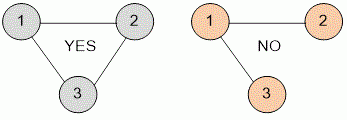Competitions

# Transitivity of a graph

Undirected graph without loops and multiple edges is called transitive, if from conditions that vertices u and v are connected with an edge, vertices v and w are connected with an edge and all three vertices u, v and w are different, implies that vertices u and w are connected with an edge.

Verify that a given undirected graph is transitive.

#### Input

The first line contains the number of vertices n and edges m of the graph (3n100, 0m ≤ (n · (n - 1)) / 2). Then m lines are given - the list of edges.

#### Output

Print YES or NO - the answer to the question about transitivity of a graph.Time limit 1 second
Memory limit 128 MiB
Input example #1
3 3
1 2
2 3
1 3

Output example #1
YES

Input example #2
3 2
1 2
1 3

Output example #2
NO